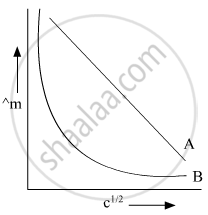Advertisement Remove all ads

# In the Plot of Molar Conductivity (∧M) Vs Square Root of Concentration (C1/2), Following Curves Are Obtained for Two Electrolytes a and B: Answer the Following: (I) Predict the Nature of Electrolytes - Chemistry

Advertisement Remove all ads
Advertisement Remove all ads
Advertisement Remove all ads
Short Note

In the plot of molar conductivity (∧m) vs square root of concentration (c1/2), following curves are obtained for two electrolytes A and B:Answer the following:
(i) Predict the nature of electrolytes A and B.
(ii) What happens on extrapolation of ∧m to concentration approaching zero for electrolytes A and B?

Advertisement Remove all ads

#### Solution

(i)
A =  Strong electrolyte
B =  Weak electrolyte

(ii) On extrapolation of these graphs they cut the Y-axis it means concentration is approaching zero and at that point, we will say infinite dilution of their respective A and B.
When A and B cut on Y-axis then we will say molar conductivity of A and B at infinite dilution.

Concept: Conductance of Electrolytic Solutions - Variation of Conductivity and Molar Conductivity with Concentration
Is there an error in this question or solution?

#### Video TutorialsVIEW ALL 

Advertisement Remove all ads
Share
Notifications

View all notifications

Forgot password?